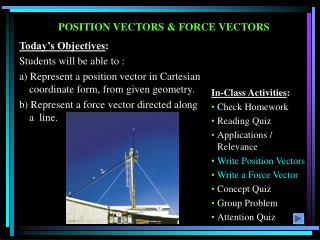DownloadDownload PresentationPOSITION VECTORS & FORCE VECTORS

# POSITION VECTORS & FORCE VECTORS

Télécharger la présentation## POSITION VECTORS & FORCE VECTORS

- - - - - - - - - - - - - - - - - - - - - - - - - - - E N D - - - - - - - - - - - - - - - - - - - - - - - - - - -
##### Presentation Transcript

1. POSITION VECTORS & FORCE VECTORS Today’s Objectives: Students will be able to : a) Represent a position vector in Cartesian coordinate form, from given geometry. b) Represent a force vector directed along a line. • In-Class Activities: • Check Homework • Reading Quiz • Applications / Relevance • Write Position Vectors • Write a Force Vector • Concept Quiz • Group Problem • Attention Quiz

2. READING QUIZ 1. A position vector, rPQ, is obtained by A) Coordinates of Q minus coordinates of P B) Coordinates of P minus coordinates of Q C) Coordinates of Q minus coordinates of the origin D) Coordinates of the origin minus coordinates of P 2. A force of magnitude F, directed along a unit vector U, is given by F = ______ . A) F (U) B) U / F C) F / U D) F + U E) F –U

3. APPLICATIONS How can we represent the force along the wing strut in a 3-D Cartesian vector form? Wing strut

4. POSITION VECTOR A position vector is defined as a fixed vector that locates a point in space relative to another point. Consider two points, A & B, in 3-D space. Let their coordinates be (XA, YA, ZA) and ( XB, YB, ZB ), respectively. The position vector directed from A to B, r AB , is defined as r AB = {( XB– XA )i + ( YB– YA ) j + ( ZB– ZA ) k }m Please note that B is the ending point and A is the starting point. So ALWAYS subtract the “tail” coordinates from the “tip” coordinates!

5. FORCE VECTOR DIRECTED ALONG A LINE (Section 2.8) If a force is directed along a line, then we can represent the force vector in Cartesian Coordinates by using a unit vector and the force magnitude. So we need to: a) Find the position vector, r AB, along two points on that line. b) Find the unit vector describing the line’s direction, uAB = (rAB/rAB). c) Multiply the unit vector by the magnitude of the force, F = F uAB .

6. EXAMPLE Given: 400 lb force along the cable DA. Find: The force FDA in the Cartesian vector form. • Plan: • Find the position vector rDA and the unit vector uDA. • 2. Obtain the force vector as FDA = 400 lb uDA .

7. EXAMPLE (continued) The figure shows that when relating D to A, we will have to go -2 ft in the x-direction, -6 ft in the y-direction, and +14 ft in the z-direction. Hence, rDA = {-2 i– 6 j + 14 k} ft. We can also find rDA by subtracting the coordinates of D from the coordinates of A. rDA = (22 + 62 + 142)0.5 = 15.36 ft uDA= rDA/rDA and FDA = 400 uDA lb FDA = 400{(-2 i– 6 j + 14 k)/15.36} lb = {-52.1 i – 156 j + 365 k} lb

8. CONCEPT QUIZ 1. P and Q are two points in a 3-D space. How are the position vectors rPQ and rQP related? A) rPQ = rQP B) rPQ = -rQP C) rPQ = 1/rQP D) rPQ = 2 rQP 2. If F and r are force vector and position vectors, respectively, in SI units, what are the units of the expression (r * (F / F)) ? A) Newton B) Dimensionless C) Meter D) Newton - Meter E) The expression is algebraically illegal.

9. GROUP PROBLEM SOLVING Given: Two forces are acting on a pipe as shown in the figure. Find: The magnitude and the coordinate direction angles of the resultant force. • Plan: • Find the forces along CA and CB in the Cartesian vector form. • 2) Add the two forces to get the resultant force, FR. • 3) Determine the magnitude and the coordinate angles of FR.

10. GROUP PROBLEM SOLVING (continued) FCA= 100 lb{rCA/rCA} FCA = 100 lb(-3 sin 40°i + 3 cos 40°j – 4 k)/5 FCA= {-38.57 i + 45.96 j – 80 k} lb FCB = 81 lb{rCB/rCB} FCB = 81 lb(4 i – 7 j – 4 k)/9 FCB = {36i– 63 j – 36 k} lb FR = FCA + FCB = {-2.57 i – 17.04 j – 116 k} lb FR = (2.572 + 17.042 + 1162) = 117.3 lb = 117 lb  = cos-1(-2.57/117.3) = 91.3°,  = cos-1(-17.04/117.3) = 98.4°  = cos-1(-116/117.3) = 172°

11. ATTENTION QUIZ 1. Two points in 3 – D space have coordinates of P (1, 2, 3) and Q (4, 5, 6) meters. The position vector rQP is given by A) {3 i + 3 j + 3 k}m B) {- 3 i– 3 j– 3 k}m C) {5 i + 7 j + 9 k} m D) {- 3 i + 3j+ 3 k} m E) {4 i + 5 j + 6 k} m 2. Force vector, F, directed along a line PQ is given by A) (F/ F) rPQ B) rPQ/rPQ C) F(rPQ/rPQ) D) F(rPQ/rPQ)

12. End of the Lecture Let Learning Continue